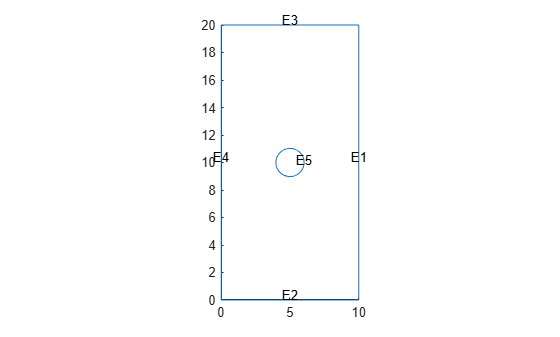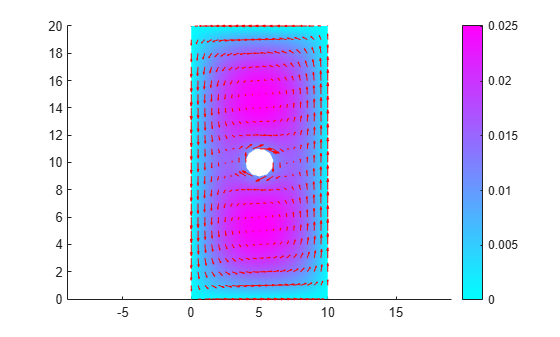Main Content

# MagnetostaticResults

Magnetostatic solution and derived quantities

## Description

A `MagnetostaticResults` object contains the magnetic potential, magnetic field, and magnetic flux density values in a form convenient for plotting and postprocessing.

The magnetic potential, magnetic field, and magnetic flux density are calculated at the nodes of the triangular or tetrahedral mesh generated by `generateMesh`. Magnetic potential values at the nodes appear in the `MagneticPotential` property. Magnetic field values at the nodes appear in the `MagneticField` property. Magnetic flux density at the nodes appear in the `MagneticFluxDensity` property.

To interpolate the magnetic potential, magnetic field, and magnetic flux density to a custom grid, such as the one specified by `meshgrid`, use the `interpolateMagneticPotential`, `interpolateMagneticField`, and `interpolateMagneticFlux` functions.

## Creation

Solve a magnetostatic problem using the `solve` function. This function returns a solution as a `MagnetostaticResults` object.

## Properties

expand all

Magnetic potential values at nodes, returned as a vector.

Data Types: `double`

Magnetic field values at nodes, returned as an `FEStruct` object. The properties of this object contain the components of the magnetic field at nodes.

Magnetic flux density values at nodes, returned as an `FEStruct` object. The properties of this object contain the components of the magnetic flux density at nodes.

Finite element mesh, returned as an FEMesh Properties object. For details, see FEMesh Properties.

## Object Functions

 `interpolateMagneticPotential` Interpolate magnetic potential in magnetostatic result at arbitrary spatial locations `interpolateMagneticField` Interpolate magnetic field in magnetostatic result at arbitrary spatial locations `interpolateMagneticFlux` Interpolate magnetic flux density in magnetostatic result at arbitrary spatial locations

## Examples

collapse all

Solve a 2-D electromagnetic problem.

Create an electromagnetic model for magnetostatic analysis.

`emagmodel = createpde('electromagnetic','magnetostatic');`

Import and plot the geometry representing a plate with a hole.

`importGeometry(emagmodel,'PlateHolePlanar.stl')`
```ans = DiscreteGeometry with properties: NumCells: 0 NumFaces: 1 NumEdges: 5 NumVertices: 5 Vertices: [5x3 double] ```
`pdegplot(emagmodel,'EdgeLabels','on')`Specify the vacuum permeability value in the SI system of units.

`emagmodel.VacuumPermeability = 1.2566370614E-6;`

Specify the relative permeability of the material.

`electromagneticProperties(emagmodel,'RelativePermeability',5000);`

Apply the magnetic potential boundary conditions on the edges framing the rectangle and the circle.

```electromagneticBC(emagmodel,'MagneticPotential',0,'Edge',1:4); electromagneticBC(emagmodel,'MagneticPotential',0.01,'Edge',5);```

Specify the current density for the entire geometry.

`electromagneticSource(emagmodel,'CurrentDensity',0.5);`

Generate the mesh.

`generateMesh(emagmodel);`

Solve the model.

`R = solve(emagmodel)`
```R = MagnetostaticResults with properties: MagneticPotential: [1218x1 double] MagneticField: [1x1 FEStruct] MagneticFluxDensity: [1x1 FEStruct] Mesh: [1x1 FEMesh] ```

Plot the magnetic potential and field.

```pdeplot(emagmodel,'XYData',R.MagneticPotential, ... 'FlowData',[R.MagneticField.Hx ... R.MagneticField.Hy]) axis equal```## See Also

Introduced in R2021a

## Support#### Try MATLAB, Simulink, and Other Products

Get trial now